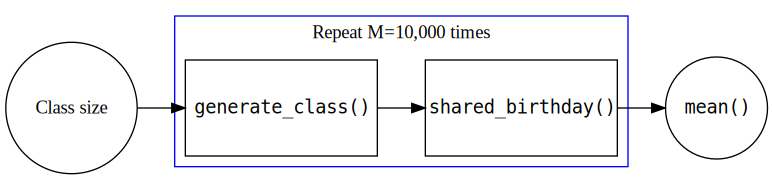## Probability

What is probability?

## Example

What do we mean by probability in the following examples?

1. In a class of 37 individuals, the probability that two students share a birthday is 0.84.

## Example

What do we mean by probability in the following examples?

1. In a class of 37 individuals, the probability that two students share a birthday is 0.84.
2. I think there is an 80% chance the defendant did the crime.

## Example

What do we mean by probability in the following examples?

1. In a class of 37 individuals, the probability that two students share a birthday is 0.84.
2. I think there is an 80% chance the defendant did the crime.
3. After performing the clinical trial, scientists reported a 0.98 probability that the treatment is effective.

## The term probability has multiple definitions.

1. In a class of 37 individuals, the probability that two students share a birthday is 0.84.
2. I think there is an 80% chance the defendant did the crime.
3. After performing the clinical trial, scientists reported a 0.98 probability that the treatment is effective.

## The term probability has multiple definitions.

1. Frequency In a class of 37 individuals, the probability that two students share a birthday is 0.84.
2. I think there is an 80% chance the defendant did the crime.
3. After performing the clinical trial, scientists reported a 0.98 probability that the treatment is effective.

## The term probability has multiple definitions.

1. Frequency. In a class of 37 individuals, the probability that two students share a birthday is 0.84.
2. Expression of personal belief. I think there is an 80% chance the defendant did the crime.
3. After performing the clinical trial, scientists reported a 0.98 probability that the treatment is effective.

## The term probability has multiple definitions.

1. Frequency. In a class of 37 individuals, the probability that two students share a birthday is 0.84.
2. Expression of personal belief. I think there is an 80% chance the defendant did the crime.
3. A combination of frequency and belief. After performing the clinical trial, scientists reported a 0.98 probability that the treatment is effective.

## Frequency definition of Probability

Key elements:

1. Repeatable process
2. Recordable outcome from each execution of the process
3. Proportion
$\frac{\text{Frequency of outcome of interest}}{\text{Total number of replicates}}$
4. Limit

## Birthday example (will revisit)

generate_class <- function(class_size){
???
???
}

check_birthday <- function(class){
???
???
}

replicates <- replicate(???, ???)
mean(replicates)

## Operating characteristic

The long-run proportion is only one of the features of the repeatable process.

## Operating characteristic

The long-run proportion is only one of the features of the repeatable process.

Key features of a procedure are often called operating characteristics.

## Example

What is the distribution of the largest run in a sequence of 100 flips of a fair coin?

## Operating characteristic

Key properties of a procedure are often called operating characteristics. Generally, one wants to know the distribution of an operating characteristic over repeated executions of the study.

## Operating characteristic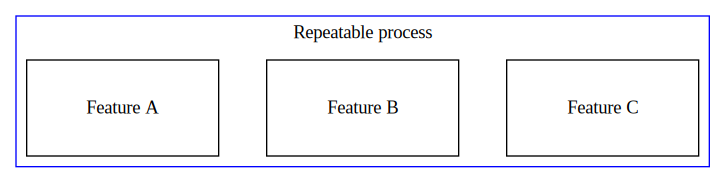## Operating characteristic

Operating characteristics are the currency by which we evaluate and compare data science procedures.

## Examples/Questions

1. A data scientist claims to have developed a tool to identify college freshman that are highly likely to join the armed forces. What operating characteristics would you like to know about the tool?

2. A data scientist develops an algorithm for estimating the probability that a credit card transaction is fraudulent or not. What operating characteristics are important?

Operating characteristics are premised on the classic “long-run” interpretation of probabilistic events. As such, they can be simulated by simply repeating the planned procedure and observing how often some event happens.

## How do we evaluate operating characteristics?

1. Analytic evaluation (we work out the mathematics and asymptotics)
2. Simulation

In this class, we are going to use both tools.

## tgs axioms of computing

So you want to perform a simulation study? Start with the tgs axioms of computing:

### Axiom 1.

The act of turning on the computer does not magically endow you with understanding of your task. If you do not know how you will perform an analysis or simulation before you turn on your computer, you will not know how to do it afterwards either.

## tgs axioms of computing

### Axiom 2.

Use modular/functional programming. Functional programming means that you identify and write short, single purpose functions for each distinct task in your program. (Examples below.) This will allow you to develop your code in a systematic way, and it will provide a natural method for debugging your code. You will simply need to verify that the different sub-functions are working as expected.

## The big picture of simulation studies for stochastic processes

In a setting where one is trying to understand a random process, identify the input parameters and the desired characteristic.

## The big picture of simulation studies for stochastic processes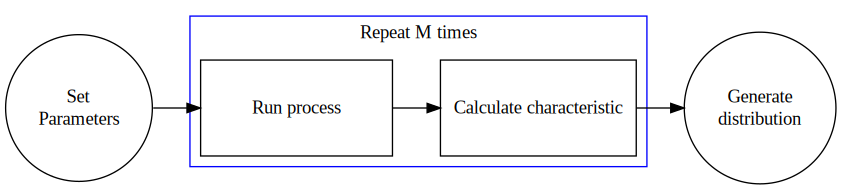## The big picture of simulation studies for inference

In a typical data analysis setting, there are population parameters that one hopes to estimate by collecting and analyzing data. The population parameters are unknown, and the accuracy of the conclusions is unknown.

## The big picture of simulation studies for inference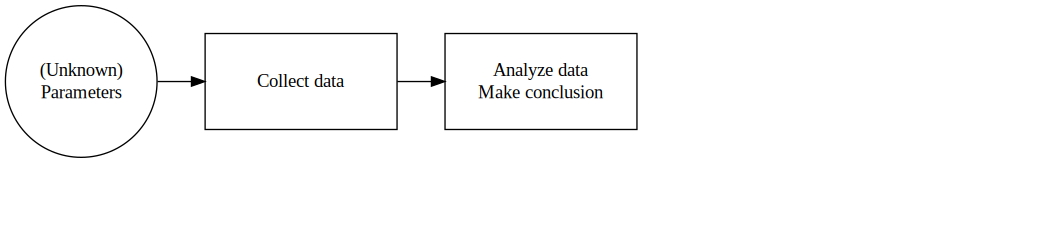## The big picture of simulation studies for inference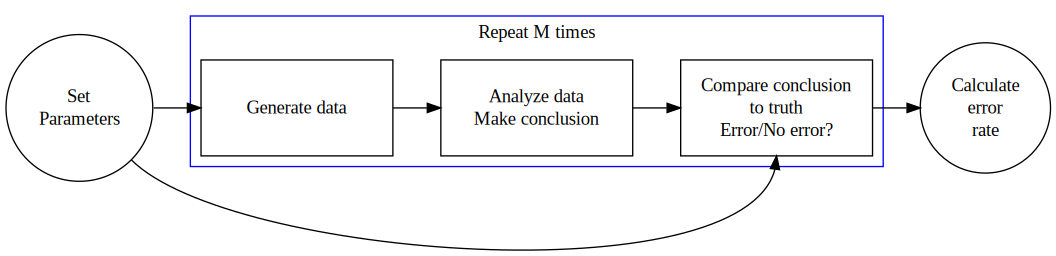## The big picture of simulation studies for inference

In a simulation setting, the researcher sets the population parameters then generates data using the parameters. After completing the analysis, the researcher can then evaluate the accuracy of the conclusions. By repeating this process several times, the researcher can estimate the operating characteristics for the specific set of population parameters used to simulate the data.

How might we change this statement so that it is applicable to prediction?

In a simulation setting, the researcher sets the population parameters then generates data using the parameters. After completing the analysis, the researcher can then evaluate the accuracy of the conclusions. By repeating this process several times, the researcher can estimate the operating characteristics for the specific set of population parameters used to simulate the data.

How might we change this statement so that it is applicable to prediction?

In a simulation setting, the researcher sets the population parameters then generates data using the parameters. After completing the analysis, the researcher can then evaluate the accuracy of the conclusions predictions. By repeating this process several times, the researcher can estimate the operating characteristics (usually call measures of model performance) for the specific set of population parameters used to simulate the data.

## Caveat emptor

Simulations are essentially computational proofs on a case-by-case basis (e.g., this result happens for this parameter set, that result happens for that parameter set). It is up to you make the conneciton between different settings, notice patterns, and make a case for general patterns of behavior under certain circumstances. Simulations lack the natural generalizability of a mathematical proof, so the results don’t necessary hold for all sets of parameter values.

## How to write a simulation studies

The framework described above suggests how one may write modular code to perform the simulation. One can write a function to perform each of the primary tasks. For example:

## How to write a simulation studies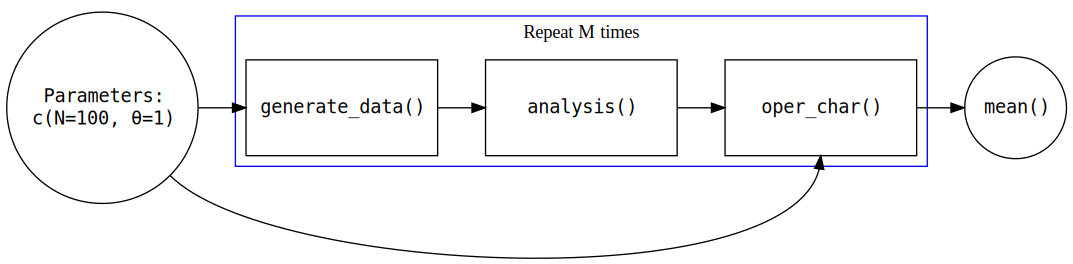## Summary

1. 3 different definitions of probability
2. The frequency definition of probability is a type of operating characteristic
3. Operating characteristics are the currency by which we evaluate and compare data science tools
4. We can evaluate operating characteristics either with analytic methods or simulation
5. Recommendations for coding simulations

## Back to the birthday example

How might we use this as a template for the birthday question?## Back to the birthday example

How might we use this as a template for the birthday question?